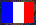Version française

# Armpi 4 - Calculating Pi

Armpi 4 is a program that calculates π in decimal for the ARM processors (in particular for the Acorn Risc PC). It is the successor of Armpi 3. Most explanations are given in the document relative to the version 3; only the changes are given here.

Like with the version 3, the assembly source is given in a separate file. Perl scripts enable to extract the assembly source from the given file in accordance with the standard syntax or the source for extASM. Another Perl script creates a BASIC program (for the Risc PC) from the assembly source returned by the first script.

Only the conversion into base 10 has been modified. There are two main changes:

• When a bit of the binary number is 0, the result of the division by 2 is useless; therefore this division is not performed, and a division by 4 will be performed at the next iteration (even if the next bit is also 0: there are never divisions by 8). The calculation time of a division by 4 is slightly less than the one of 2 divisions by 2. But, in order to be fast, the division by 4 is performed with a redundant representation, the digits being between 0 and 27, and the cleaning of the result must be performed twice as often, but globally the calculation is really faster. Note that the division by 2 remains valid.
• The cleaning of the result is performed every 4 additions instead of every 4 read bits (or every 8 read bits with Armpi 3, where there is no division by 4).

The division by 4 is performed a little like the division by 2, except the backcarries (so called because they correspond to carries in the multiplication by 4). When a digit (between 0 and 27) is divided by 4, a quotient between 0 and 6 and a remainder between 0 and 3 are obtained. A remainder equal to r generates two backcarries respectively equal to 2r and to 5r for the next two digits. Thus a value up to 21 is added to each digit, so one verifies that the digits can't be higher than 27. Concerning the implementation, the backcarries are added sometimes before, sometimes after the division of the digit by 4 (in the former case, the backcarries are obviously multiplied by 4), and the code is particularly optimised; the best way to see how it works is to take examples and to run the code on them...

## Results and Comparisons

200,000 decimals have been calculated in 6 hours 54 minutes 4 seconds: the binary calculation has lasted 3 hours 47 minutes 12 seconds and the conversion into base 10 has lasted 3 hours 6 minutes 52 seconds.

The comparisons with other computers and other π calculation programs I've written are given in a separate file; all the programs up to Armpi 4 use the same formula, but the algorithms are quite different.Last updated:
• XML source: 2003-06-18, 23:39:56 (20)
• XSL stylesheet: 2003-08-06, 22:11:54 (488)
webmaster@vinc17.org